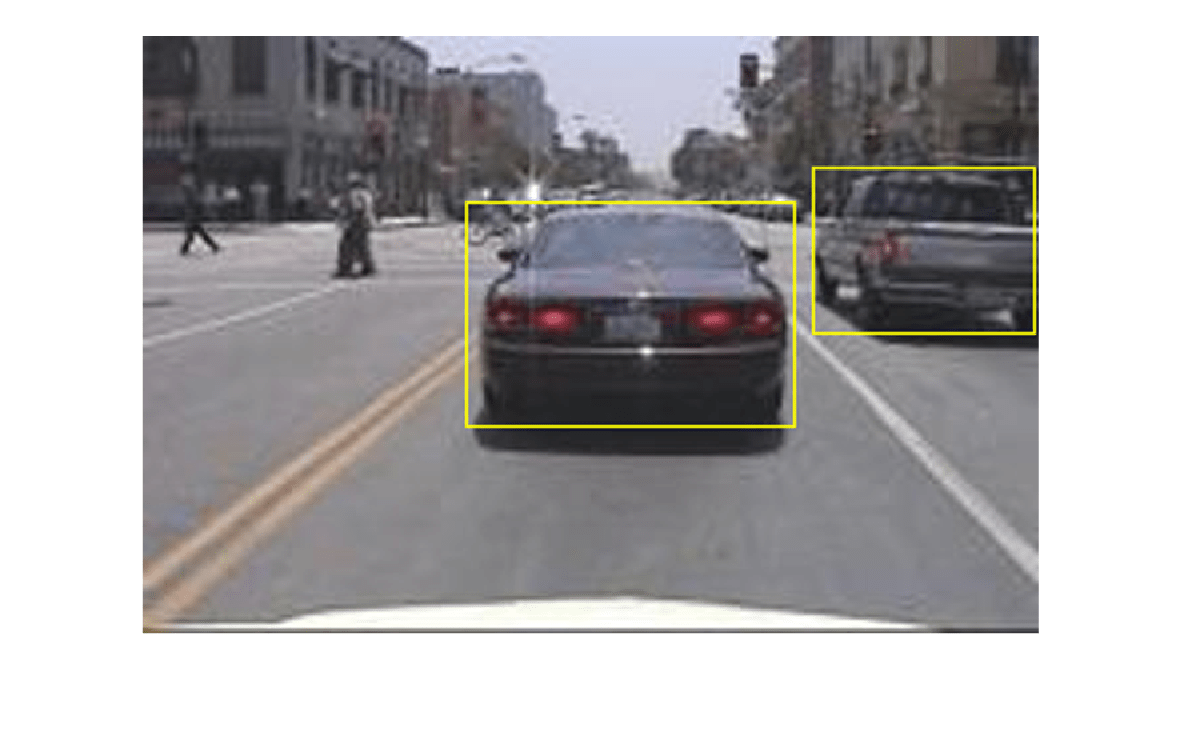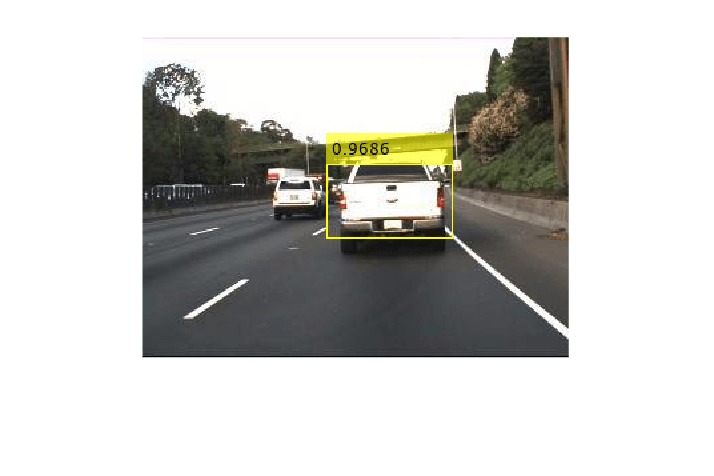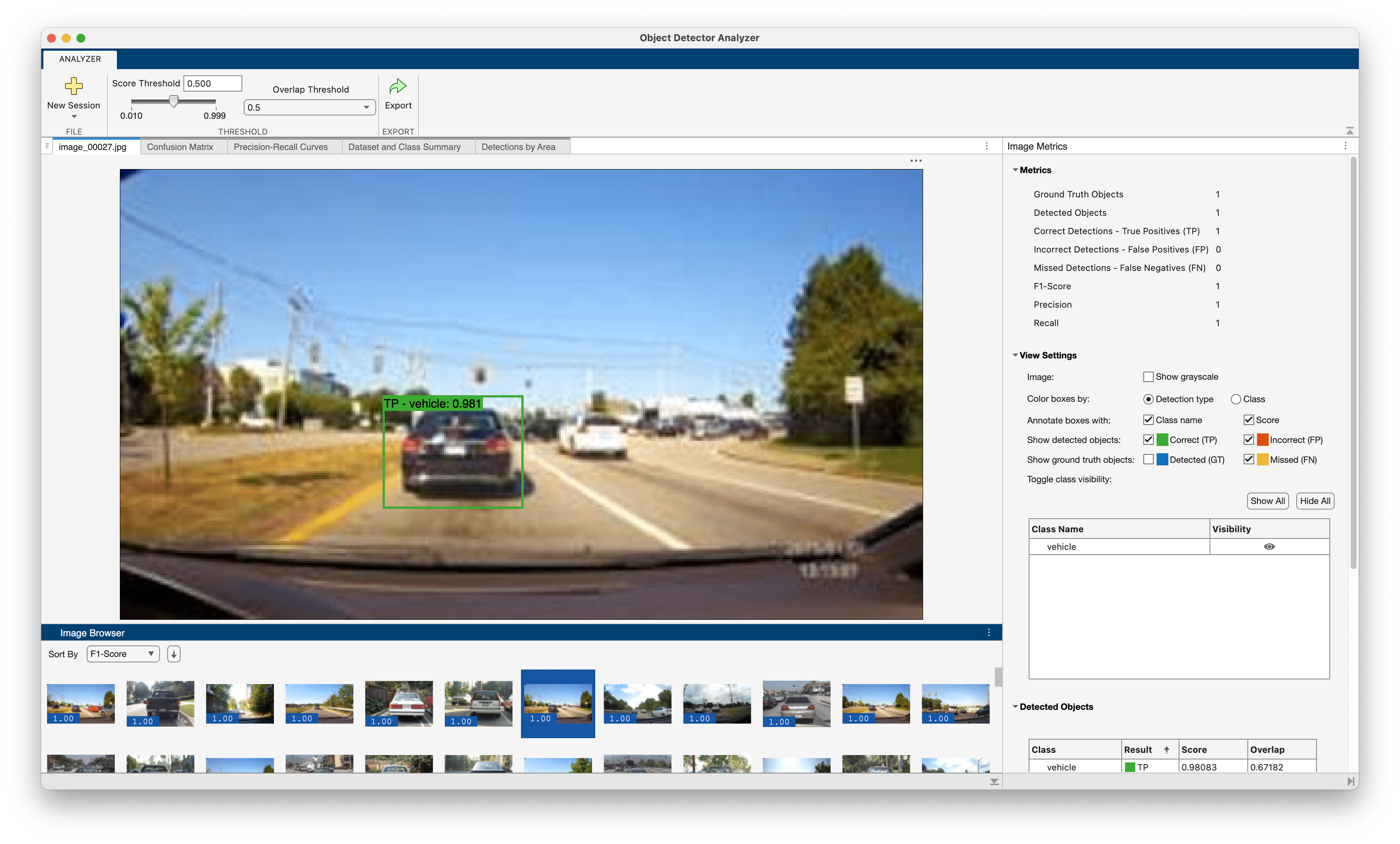# Object Detection Using YOLO v4 Deep Learning

This example shows how to detect objects in images using you only look once version 4 (YOLO v4) deep learning network. In this example, you will

• Configure a dataset for training, validation, and testing of YOLO v4 object detection network. You will also perform data augmentation on the training dataset to improve the network efficiency.

• Compute anchor boxes from the training data to use for training the YOLO v4 object detection network.

• Create a YOLO v4 object detector by using the `yolov4ObjectDetector` function and train the detector using `trainYOLOv4ObjectDetector` function.

This example also provides a pretrained YOLO v4 object detector to use for detecting vehicles in an image. The pretrained network uses CSPDarkNet-53 as the backbone network and is trained on a vehicle dataset. For information about YOLO v4 object detection network, see Getting Started with YOLO v4.

This example uses a small vehicle dataset that contains 295 images. Many of these images come from the Caltech Cars 1999 and 2001 datasets, available at the Caltech Computational Vision website created by Pietro Perona and used with permission. Each image contain one or two labeled instances of a vehicle. A small dataset is useful for exploring the YOLO v4 training procedure, but in practice, more labeled images are needed to train a robust detector.

Unzip the vehicle images and load the vehicle ground truth data.

```unzip vehicleDatasetImages.zip data = load("vehicleDatasetGroundTruth.mat"); vehicleDataset = data.vehicleDataset;```

The vehicle data is stored in a two-column table. The first column contain the image file paths and the second column contain the bounding boxes.

```% Display first few rows of the data set. vehicleDataset(1:4,:)```
```ans=4×2 table imageFilename vehicle _________________________________ _________________ {'vehicleImages/image_00001.jpg'} {[220 136 35 28]} {'vehicleImages/image_00002.jpg'} {[175 126 61 45]} {'vehicleImages/image_00003.jpg'} {[108 120 45 33]} {'vehicleImages/image_00004.jpg'} {[124 112 38 36]} ```
```% Add the fullpath to the local vehicle data folder. vehicleDataset.imageFilename = fullfile(pwd,vehicleDataset.imageFilename);```

Split the dataset into training, validation, and test sets. Select 60% of the data for training, 10% for validation, and the rest for testing the trained detector.

```rng("default"); shuffledIndices = randperm(height(vehicleDataset)); idx = floor(0.6 * length(shuffledIndices) ); trainingIdx = 1:idx; trainingDataTbl = vehicleDataset(shuffledIndices(trainingIdx),:); validationIdx = idx+1 : idx + 1 + floor(0.1 * length(shuffledIndices) ); validationDataTbl = vehicleDataset(shuffledIndices(validationIdx),:); testIdx = validationIdx(end)+1 : length(shuffledIndices); testDataTbl = vehicleDataset(shuffledIndices(testIdx),:);```

Use `imageDatastore` and `boxLabelDatastore` to create datastores for loading the image and label data during training and evaluation.

```imdsTrain = imageDatastore(trainingDataTbl{:,"imageFilename"}); bldsTrain = boxLabelDatastore(trainingDataTbl(:,"vehicle")); imdsValidation = imageDatastore(validationDataTbl{:,"imageFilename"}); bldsValidation = boxLabelDatastore(validationDataTbl(:,"vehicle")); imdsTest = imageDatastore(testDataTbl{:,"imageFilename"}); bldsTest = boxLabelDatastore(testDataTbl(:,"vehicle"));```

Combine image and box label datastores.

```trainingData = combine(imdsTrain,bldsTrain); validationData = combine(imdsValidation,bldsValidation); testData = combine(imdsTest,bldsTest);```

Use `validateInputData` to detect invalid images, bounding boxes or labels i.e.,

• Samples with invalid image format or containing NaNs

• Bounding boxes containing zeros/NaNs/Infs/empty

• Missing/non-categorical labels.

The values of the bounding boxes must be finite positive integers and must not be NaN. The height and the width of the bounding box values must be positive and lie within the image boundary.

```validateInputData(trainingData); validateInputData(validationData); validateInputData(testData);```

Display one of the training images and box labels.

```data = read(trainingData); I = data{1}; bbox = data{2}; annotatedImage = insertShape(I,"Rectangle",bbox); annotatedImage = imresize(annotatedImage,2); figure imshow(annotatedImage)````reset(trainingData);`

### Create a YOLO v4 Object Detector Network

Specify the network input size to be used for training.

`inputSize = [608 608 3];`

Specify the name of the object class to detect.

`className = "vehicle";`

Use the `estimateAnchorBoxes` function to estimate anchor boxes based on the size of objects in the training data. To account for the resizing of the images prior to training, resize the training data for estimating anchor boxes. Use `transform` to preprocess the training data, then define the number of anchor boxes and estimate the anchor boxes. Resize the training data to the input size of the network by using the `preprocessData` helper function.

```rng("default") trainingDataForEstimation = transform(trainingData,@(data)preprocessData(data,inputSize)); numAnchors = 9; [anchors,meanIoU] = estimateAnchorBoxes(trainingDataForEstimation,numAnchors); area = anchors(:, 1).*anchors(:,2); [~,idx] = sort(area,"descend"); anchors = anchors(idx,:); anchorBoxes = {anchors(1:3,:) anchors(4:6,:) anchors(7:9,:) };```

For more information on choosing anchor boxes, see Estimate Anchor Boxes From Training Data (Computer Vision Toolbox™) and Anchor Boxes for Object Detection.

Create the YOLO v4 object detector by using the `yolov4ObjectDetector` function. specify the name of the pretrained YOLO v4 detection network trained on COCO dataset. Specify the class name and the estimated anchor boxes.

`detector = yolov4ObjectDetector("csp-darknet53-coco",className,anchorBoxes,InputSize=inputSize);`

### Perform Data Augmentation

Perform data augmentation to improve training accuracy. Use the `transform` function to apply custom data augmentations to the training data. The `augmentData` helper function applies the following augmentations to the input data:

• Color jitter augmentation in HSV space

• Random horizontal flip

• Random scaling by 10 percent

Note that data augmentation is not applied to the test and validation data. Ideally, test and validation data should be representative of the original data and is left unmodified for unbiased evaluation.

`augmentedTrainingData = transform(trainingData,@augmentData);`

Read and display samples of augmented training data.

```augmentedData = cell(4,1); for k = 1:4 data = read(augmentedTrainingData); augmentedData{k} = insertShape(data{1},"rectangle",data{2}); reset(augmentedTrainingData); end figure montage(augmentedData,BorderSize=10)```### Specify Training Options

Use `trainingOptions` to specify network training options. Train the object detector using the Adam solver for 70 epochs with a constant learning rate 0.001. "`ResetInputNormalization"` should be set to false and "`BatchNormalizationStatistics"` should be set to "`moving"`. Set "`ValidationData"` to the validation data and "`ValidationFrequency`" to 1000. To validate the data more often, you can reduce the “`ValidationFrequency`” which also increases the training time. Use "`ExecutionEnvironment"` to determine what hardware resources will be used to train the network. Default value for this is "auto" which selects a GPU if it is available, otherwise selects the CPU. Set "`CheckpointPath"` to a temporary location. This enables the saving of partially trained detectors during the training process. If training is interrupted, such as by a power outage or system failure, you can resume training from the saved checkpoint.

```options = trainingOptions("adam",... GradientDecayFactor=0.9,... SquaredGradientDecayFactor=0.999,... InitialLearnRate=0.001,... LearnRateSchedule="none",... MiniBatchSize=4,... L2Regularization=0.0005,... MaxEpochs=70,... BatchNormalizationStatistics="moving",... DispatchInBackground=true,... ResetInputNormalization=false,... Shuffle="every-epoch",... VerboseFrequency=20,... ValidationFrequency=1000,... CheckpointPath=tempdir,... ValidationData=validationData);```

### Train YOLO v4 Object Detector

Use the `trainYOLOv4ObjectDetector` function to train YOLO v4 object detector. This example is run on an NVIDIA™ Titan RTX GPU with 24 GB of memory. Training this network took approximately 6 hours using this setup. The training time will vary depending on the hardware you use. Instead of training the network, you can also use a pretrained YOLO v4 object detector in the Computer Vision Toolbox ™.

Download the pretrained detector by using the `downloadPretrainedYOLOv4Detector` helper function. Set the `doTraining` value to false. If you want to train the detector on the augmented training data, set the `doTraining` value to true.

```doTraining = false; if doTraining % Train the YOLO v4 detector. [detector,info] = trainYOLOv4ObjectDetector(augmentedTrainingData,detector,options); else % Load pretrained detector for the example. detector = downloadPretrainedYOLOv4Detector(); end```

Run the detector on a test image.

```I = imread("highway.png"); [bboxes,scores,labels] = detect(detector,I);```

Display the results.

```I = insertObjectAnnotation(I,"rectangle",bboxes,scores); figure imshow(I)```### Evaluate Detector Using Test Set

Evaluate the trained object detector on a large set of images to measure the performance. Computer Vision Toolbox™ provides object detector evaluation functions to measure common metrics such as average precision (`evaluateDetectionPrecision`) and log-average miss rates (`evaluateDetectionMissRate`). For this example, use the average precision metric to evaluate performance. The average precision provides a single number that incorporates the ability of the detector to make correct classifications (precision) and the ability of the detector to find all relevant objects (recall).

Run the detector on all the test images.

`detectionResults = detect(detector,testData);`

Evaluate the object detector using average precision metric.

`[ap,recall,precision] = evaluateDetectionPrecision(detectionResults,testData);`

The precision/recall (PR) curve highlights how precise a detector is at varying levels of recall. The ideal precision is 1 at all recall levels. The use of more data can help improve the average precision but might require more training time. Plot the PR curve.

```figure plot(recall,precision) xlabel("Recall") ylabel("Precision") grid on title(sprintf("Average Precision = %.2f",ap))```### Supporting Functions

Helper function for performing data augmentation.

```function data = augmentData(A) % Apply random horizontal flipping, and random X/Y scaling. Boxes that get % scaled outside the bounds are clipped if the overlap is above 0.25. Also, % jitter image color. data = cell(size(A)); for ii = 1:size(A,1) I = A{ii,1}; bboxes = A{ii,2}; labels = A{ii,3}; sz = size(I); if numel(sz) == 3 && sz(3) == 3 I = jitterColorHSV(I,... contrast=0.0,... Hue=0.1,... Saturation=0.2,... Brightness=0.2); end % Randomly flip image. tform = randomAffine2d(XReflection=true,Scale=[1 1.1]); rout = affineOutputView(sz,tform,BoundsStyle="centerOutput"); I = imwarp(I,tform,OutputView=rout); % Apply same transform to boxes. [bboxes,indices] = bboxwarp(bboxes,tform,rout,OverlapThreshold=0.25); labels = labels(indices); % Return original data only when all boxes are removed by warping. if isempty(indices) data(ii,:) = A(ii,:); else data(ii,:) = {I,bboxes,labels}; end end end function data = preprocessData(data,targetSize) % Resize the images and scale the pixels to between 0 and 1. Also scale the % corresponding bounding boxes. for ii = 1:size(data,1) I = data{ii,1}; imgSize = size(I); bboxes = data{ii,2}; I = im2single(imresize(I,targetSize(1:2))); scale = targetSize(1:2)./imgSize(1:2); bboxes = bboxresize(bboxes,scale); data(ii,1:2) = {I,bboxes}; end end```

```function detector = downloadPretrainedYOLOv4Detector() % Download a pretrained yolov4 detector. if ~exist("yolov4CSPDarknet53VehicleExample_22a.mat", "file") if ~exist("yolov4CSPDarknet53VehicleExample_22a.zip", "file") disp("Downloading pretrained detector..."); pretrainedURL = "https://ssd.mathworks.com/supportfiles/vision/data/yolov4CSPDarknet53VehicleExample_22a.zip"; websave("yolov4CSPDarknet53VehicleExample_22a.zip", pretrainedURL); end unzip("yolov4CSPDarknet53VehicleExample_22a.zip"); end pretrained = load("yolov4CSPDarknet53VehicleExample_22a.mat"); detector = pretrained.detector; end```